Documentation

# findchangepts

Find abrupt changes in signal

## Syntax

``ipt = findchangepts(x)``
``ipt = findchangepts(x,Name,Value)``
``````[ipt,residual] = findchangepts(___)``````
``findchangepts(___)``

## Description

example

````ipt = findchangepts(x)` returns the index at which the mean of `x` changes most significantly. If `x` is a vector with N elements, then `findchangepts` partitions `x` into two regions, `x(1:ipt-1)` and `x(ipt:N)`, that minimize the sum of the residual (squared) error of each region from its local mean.If `x` is an M-by-N matrix, then `findchangepts` partitions `x` into two regions, `x(1:M,1:ipt-1)` and `x(1:M,ipt:N)`, returning the column index that minimizes the sum of the residual error of each region from its local M-dimensional mean. ```

example

````ipt = findchangepts(x,Name,Value)` specifies additional options using name-value pair arguments. Options include the number of changepoints to report and the statistic to measure instead of the mean. See Changepoint Detection for more information.```

example

``````[ipt,residual] = findchangepts(___)``` also returns the residual error of the signal against the modeled changes, incorporating any of the previous specifications.```

example

````findchangepts(___)` without output arguments plots the signal and any detected changepoints. See 'Statistic' for more information.```

## Examples

collapse all

Load a data file containing a recording of a train whistle sampled at 8192 Hz. Find the 10 points at which the root-mean-square level of the signal changes most significantly.

```load train findchangepts(y,'MaxNumChanges',10,'Statistic','rms')```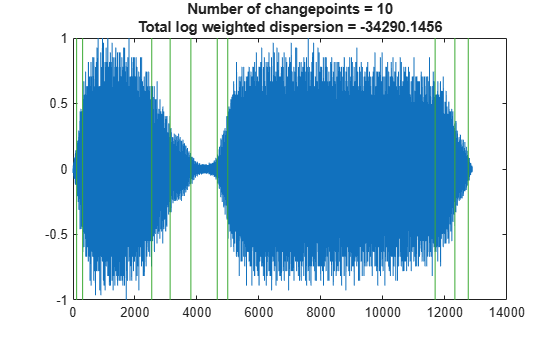Compute the short-time power spectral density of the signal. Divide the signal into 128-sample segments and window each segment with a Hamming window. Specify 120 samples of overlap between adjoining segments and 128 DFT points. Find the 10 points at which the mean of the power spectral density changes the most significantly.

```[s,f,t,pxx] = spectrogram(y,128,120,128,Fs); findchangepts(pow2db(pxx),'MaxNumChanges',10)```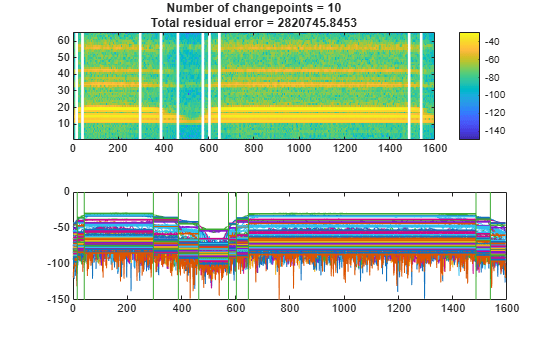Reset the random number generator for reproducible results. Generate a random signal where:

• The mean is constant in each of seven regions and changes abruptly from region to region.

• The variance is constant in each of five regions and changes abruptly from region to region.

```rng('default') lr = 20; mns = [0 1 4 -5 2 0 1]; nm = length(mns); vrs = [1 4 6 1 3]; nv = length(vrs); v = randn(1,lr*nm*nv)/2; f = reshape(repmat(mns,lr*nv,1),1,lr*nm*nv); y = reshape(repmat(vrs,lr*nm,1),1,lr*nm*nv); t = v.*y+f;```

Plot the signal, highlighting the steps of its construction.

```subplot(2,2,1) plot(v) title('Original') xlim([0 700]) subplot(2,2,2) plot([f;v+f]') title('Means') xlim([0 700]) subplot(2,2,3) plot([y;v.*y]') title('Variances') xlim([0 700]) subplot(2,2,4) plot(t) title('Final') xlim([0 700])```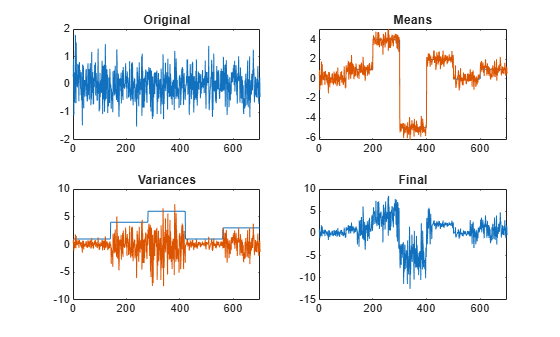Find the five points where the mean of the signal changes most significantly.

```figure findchangepts(t,'MaxNumChanges',5)```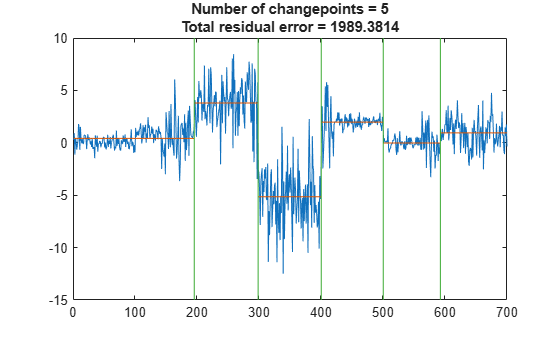Find the five points where the root-mean-square level of the signal changes most significantly.

`findchangepts(t,'MaxNumChanges',5,'Statistic','rms')`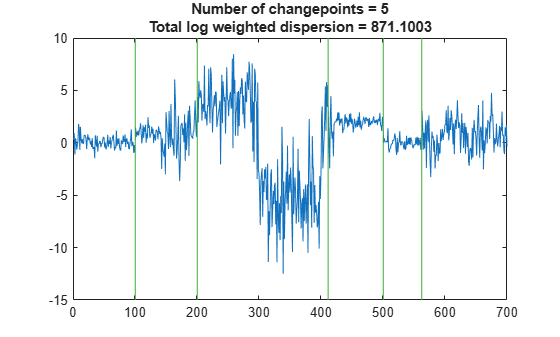Find the point where the mean and standard deviation of the signal change the most.

`findchangepts(t,'Statistic','std')`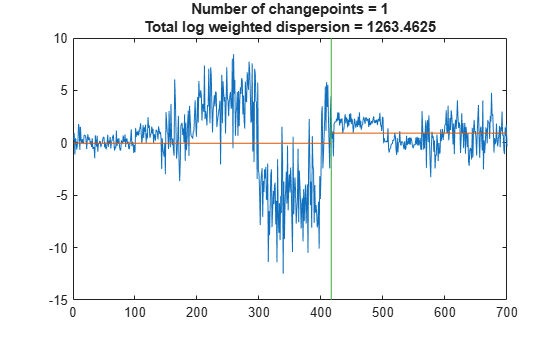Load a speech signal sampled at ${F}_{s}=7418\phantom{\rule{0.2777777777777778em}{0ex}}Hz$. The file contains a recording of a female voice saying the word "MATLAB®."

`load mtlb`

Discern the vowels and consonants in the word by finding the points at which the variance of the signal changes significantly. Limit the number of changepoints to five.

```numc = 5; [q,r] = findchangepts(mtlb,'Statistic','rms','MaxNumChanges',numc)```
```q = 5×1 132 778 1646 2500 3454 ```
```r = -4.4055e+03 ```

Plot the signal and display the changepoints.

`findchangepts(mtlb,'Statistic','rms','MaxNumChanges',numc)`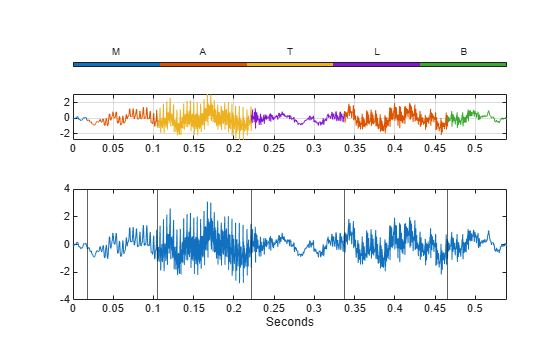To play the sound with a pause after each of the segments, uncomment the following lines.

```% soundsc(1:q(1),Fs) % for k = 1:length(q)-1 % soundsc(mtlb(q(k):q(k+1)),Fs) % pause(1) % end % soundsc(q(end):length(mtlb),Fs)```

Create a signal that consists of two sinusoids with varying amplitude and a linear trend.

```vc = sin(2*pi*(0:201)/17).*sin(2*pi*(0:201)/19).* ... [sqrt(0:0.01:1) (1:-0.01:0).^2]+(0:201)/401;```

Find the points where the signal mean changes most significantly. The `'Statistic'` name-value pair is optional in this case. Specify a minimum residual error improvement of 1.

`findchangepts(vc,'Statistic','mean','MinThreshold',1)`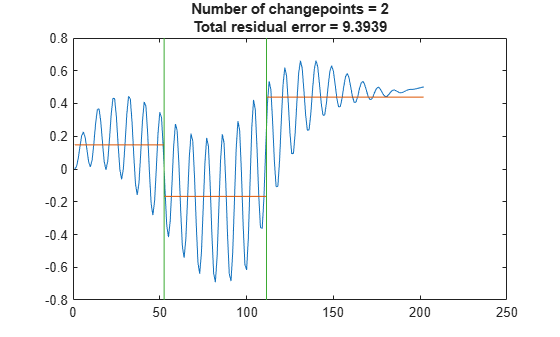Find the points where the root-mean-square level of the signal changes the most. Specify a minimum residual error improvement of 6.

`findchangepts(vc,'Statistic','rms','MinThreshold',6)`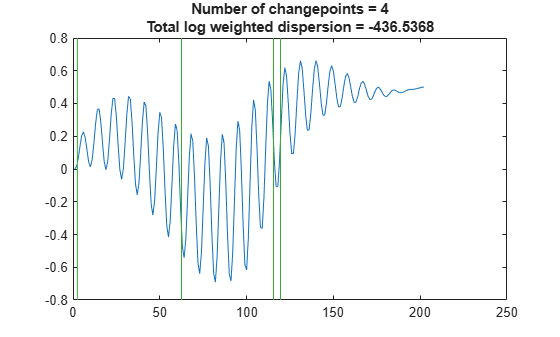Find the points where the standard deviation of the signal changes most significantly. Specify a minimum residual error improvement of 10.

`findchangepts(vc,'Statistic','std','MinThreshold',10)`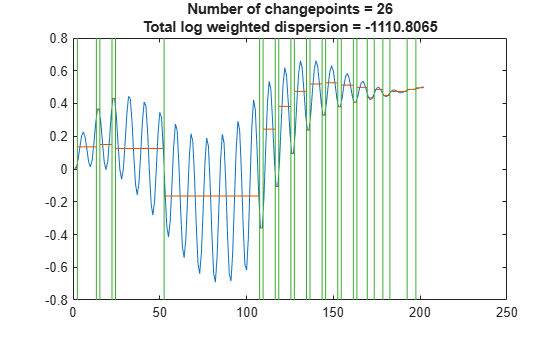Find the points where the mean and the slope of the signal change most abruptly. Specify a minimum residual error improvement of 0.6.

`findchangepts(vc,'Statistic','linear','MinThreshold',0.6)`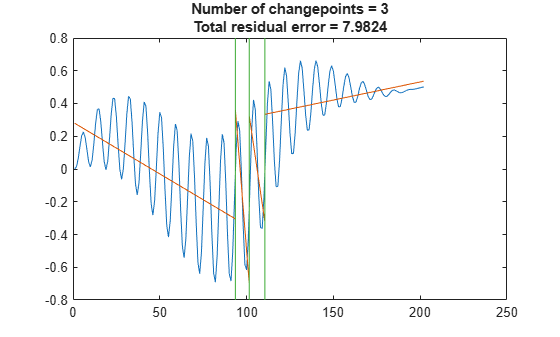Generate a two-dimensional, 1000-sample Bézier curve with 20 random control points. A Bézier curve is defined by:

$C\left(t\right)=\sum _{k=0}^{m}\left(\genfrac{}{}{0}{}{m}{k}\right){t}^{k}\left(1-t{\right)}^{m-k}{P}_{k}$,

where ${\mathit{P}}_{\mathit{k}}$ is the $\mathit{k}$th of $\mathit{m}$ control points, $\mathit{t}$ ranges from 0 to 1, and $\left(\genfrac{}{}{0}{}{m}{k}\right)$ is a binomial coefficient. Plot the curve and the control points.

```m = 20; P = randn(m,2); t = linspace(0,1,1000)'; pol = t.^(0:m-1).*(1-t).^(m-1:-1:0); bin = gamma(m)./gamma(1:m)./gamma(m:-1:1); crv = bin.*pol*P; plot(crv(:,1),crv(:,2),P(:,1),P(:,2),'o:')```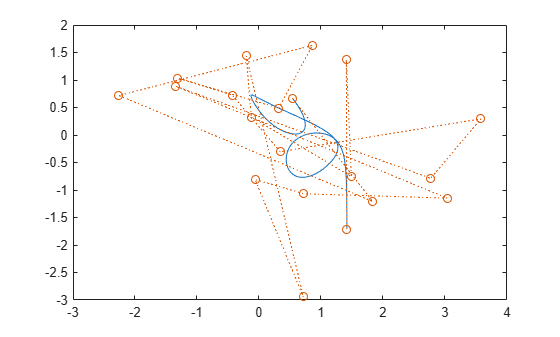Partition the curve into three segments, such that the points in each segment are at a minimum distance from the segment mean.

`findchangepts(crv','MaxNumChanges',3)`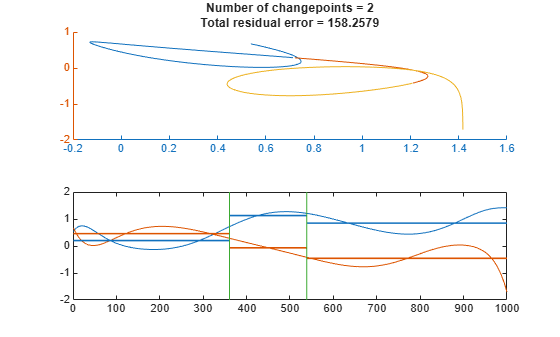Partition the curve into 20 segments that are best fit by straight lines.

`findchangepts(crv','Statistic','linear','MaxNumChanges',19)`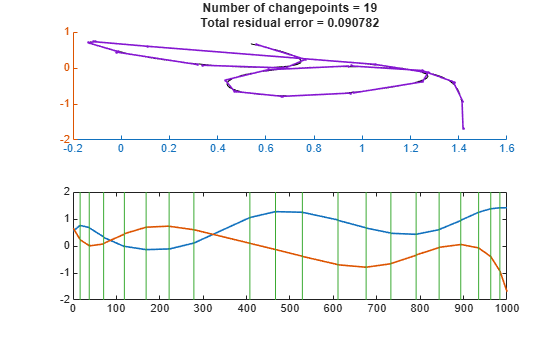Generate and plot a three-dimensional Bézier curve with 20 random control points.

```P = rand(m,3); crv = bin.*pol*P; plot3(crv(:,1),crv(:,2),crv(:,3),P(:,1),P(:,2),P(:,3),'o:') xlabel('x') ylabel('y')```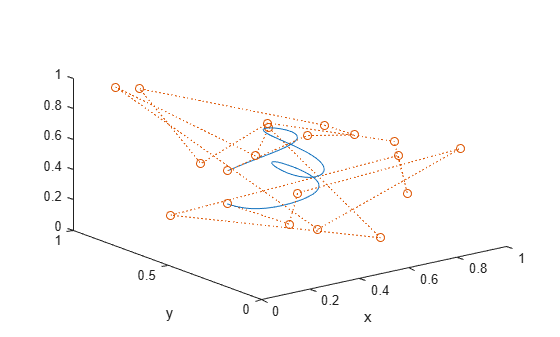Visualize the curve from above.

`view([0 0 1])`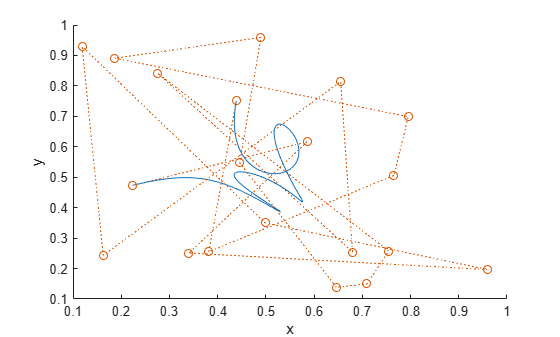Partition the curve into three segments, such that the points in each segment are at a minimum distance from the segment mean.

`findchangepts(crv','MaxNumChanges',3)`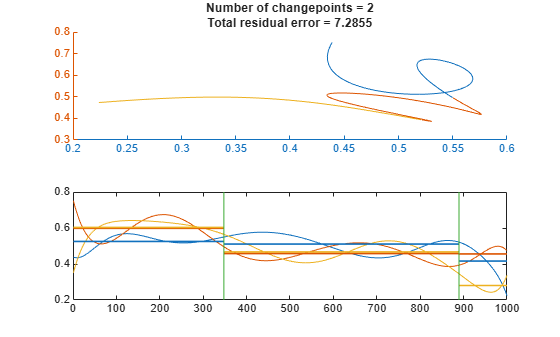Partition the curve into 20 segments that are best fit by straight lines.

`findchangepts(crv','Statistic','linear','MaxNumChanges',19)`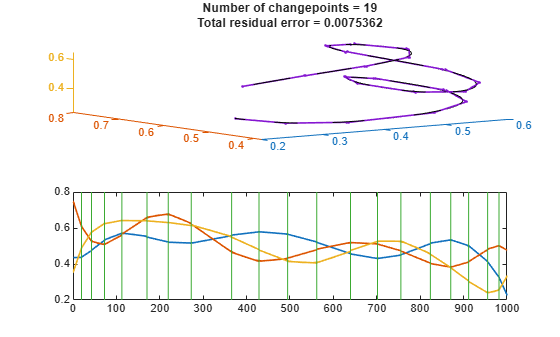## Input Arguments

collapse all

Input signal, specified as a real vector.

Example: `reshape(randn(100,3)+[-3 0 3],1,300)` is a random signal with two abrupt changes in mean.

Example: `reshape(randn(100,3).*[1 20 5],1,300)` is a random signal with two abrupt changes in root-mean-square level.

Data Types: `single` | `double`

### Name-Value Pair Arguments

Specify optional comma-separated pairs of `Name,Value` arguments. `Name` is the argument name and `Value` is the corresponding value. `Name` must appear inside quotes. You can specify several name and value pair arguments in any order as `Name1,Value1,...,NameN,ValueN`.

Example: `'MaxNumChanges',3,'Statistic','rms','MinDistance',20` finds up to three points where the changes in root-mean-square level are most significant and where the points are separated by at least 20 samples.

Maximum number of significant changes to return, specified as the comma-separated pair consisting of `'MaxNumChanges'` and an integer scalar. After finding the point with the most significant change, `findchangepts` gradually loosens its search criterion to include more changepoints without exceeding the specified maximum. If any search setting returns more than the maximum, then the function returns nothing. If `'MaxNumChanges'` is not specified, then the function returns the point with the most significant change. You cannot specify `'MinThreshold'` and `'MaxNumChanges'` simultaneously.

Example: `findchangepts([0 1 0])` returns the index of the second sample.

Example: ```findchangepts([0 1 0],'MaxNumChanges',1)``` returns an empty matrix.

Example: `findchangepts([0 1 0],'MaxNumChanges',2)` returns the indices of the second and third points.

Data Types: `single` | `double`

Type of change to detect, specified as the comma-separated pair consisting of `'Statistic'` and one of these values:

• `'mean'` — Detect changes in mean. If you call `findchangepts` with no output arguments, the function plots the signal, the changepoints, and the mean value of each segment enclosed by consecutive changepoints.

• `'rms'` — Detect changes in root-mean-square level. If you call `findchangepts` with no output arguments, the function plots the signal and the changepoints.

• `'std'` — Detect changes in standard deviation, using Gaussian log-likelihood. If you call `findchangepts` with no output arguments, the function plots the signal, the changepoints, and the mean value of each segment enclosed by consecutive changepoints.

• `'linear'` — Detect changes in mean and slope. If you call `findchangepts` with no output arguments, the function plots the signal, the changepoints, and the line that best fits each portion of the signal enclosed by consecutive changepoints.

Example: `findchangepts([0 1 2 1],'Statistic','mean')` returns the index of the second sample.

Example: ```findchangepts([0 1 2 1],'Statistic','rms')``` returns the index of the third sample.

Minimum number of samples between changepoints, specified as the comma-separated pair consisting of `'MinDistance'` and an integer scalar. If you do not specify this number, then the default is 1 for changes in mean and 2 for other changes.

Example: `findchangepts(sin(2*pi*(0:10)/5),'MaxNumChanges',5,'MinDistance',1)` returns five indices.

Example: `findchangepts(sin(2*pi*(0:10)/5),'MaxNumChanges',5,'MinDistance',3)` returns two indices.

Example: `findchangepts(sin(2*pi*(0:10)/5),'MaxNumChanges',5,'MinDistance',5)` returns no indices.

Data Types: `single` | `double`

Minimum improvement in total residual error for each changepoint, specified as the comma-separated pair consisting of `'MinThreshold'` and a real scalar that represents a penalty. This option acts to limit the number of returned significant changes by applying the additional penalty to each prospective changepoint. You cannot specify `'MinThreshold'` and `'MaxNumChanges'` simultaneously.

Example: `findchangepts([0 1 2],'MinThreshold',0)` returns two indices.

Example: ```findchangepts([0 1 2],'MinThreshold',1)``` returns one index.

Example: `findchangepts([0 1 2],'MinThreshold',2)` returns no indices.

Data Types: `single` | `double`

## Output Arguments

collapse all

Changepoint locations, returned as a vector of integer indices.

Residual error of the signal against the modeled changes, returned as a vector.

collapse all

### Changepoint Detection

A changepoint is a sample or time instant at which some statistical property of a signal changes abruptly. The property in question can be the mean of the signal, its variance, or a spectral characteristic, among others.

To find a signal changepoint, `findchangepts` employs a parametric global method. The function:

1. Chooses a point and divides the signal into two sections.

2. Computes an empirical estimate of the desired statistical property for each section.

3. At each point within a section, measures how much the property deviates from the empirical estimate. Adds the deviations for all points.

4. Adds the deviations section-to-section to find the total residual error.

5. Varies the location of the division point until the total residual error attains a minimum.

The procedure is clearest when the chosen statistic is the mean. In that case, `findchangepts` minimizes the total residual error from the "best" horizontal level for each section. Given a signal x1, x2, …, xN, the function finds k such that

`$\begin{array}{c}J=\sum _{i=1}^{k-1}{\left({x}_{i}-{〈x〉}_{1}^{k-1}\right)}^{2}+\sum _{i=k}^{N}{\left({x}_{i}-{〈x〉}_{k}^{N}\right)}^{2}\\ =\sum _{i=1}^{k-1}{\left({x}_{i}-\frac{1}{k-1}\sum _{r=1}^{k-1}{x}_{r}\right)}^{2}+\sum _{i=k}^{N}{\left({x}_{i}-\frac{1}{N-k+1}\sum _{r=k}^{N}{x}_{r}\right)}^{2}\\ =\left(k-1\right)\mathrm{var}\left(\left[\begin{array}{ccc}{x}_{1}& \cdots & {x}_{k-1}\end{array}\right]\right)+\left(N-k+1\right)\mathrm{var}\left(\left[\begin{array}{ccc}{x}_{k}& \cdots & {x}_{N}\end{array}\right]\right),\end{array}$`

is smallest. The mean and variance have the usual definitions. (The variance is normalized by the number of samples, without subtracting 1.)

This result can be generalized to incorporate other statistics. `findchangepts` finds k such that

`$J\left(k\right)=\sum _{i=1}^{k-1}\Delta \left({x}_{i};\chi \left(\left[\begin{array}{ccc}{x}_{1}& \cdots & {x}_{k-1}\end{array}\right]\right)\right)+\sum _{i=k}^{N}\Delta \left({x}_{i};\chi \left(\left[\begin{array}{ccc}{x}_{k}& \cdots & {x}_{N}\end{array}\right]\right)\right)$`

is smallest, given the section empirical estimate χ and the deviation measurement Δ. Minimizing the residual error is equivalent to maximizing the log likelihood. Given a normal distribution with mean μ and variance σ2, the log-likelihood for N independent observations is

`$\mathrm{log}\prod _{i=1}^{N}\frac{1}{\sqrt{2\pi {\sigma }^{2}}}{e}^{-{\left({x}_{i}-\mu \right)}^{2}/2{\sigma }^{2}}=-\frac{N}{2}\left(\mathrm{log}2\pi +\mathrm{log}{\sigma }^{2}\right)-\frac{1}{2{\sigma }^{2}}\sum _{i=1}^{N}{\left({x}_{i}-\mu \right)}^{2}.$`

• If `'Statistic'` is specified as `'mean'`, then the variance is fixed and the function uses

`$\begin{array}{c}\sum _{i=m}^{n}\Delta \left({x}_{i};\chi \left(\left[\begin{array}{ccc}{x}_{m}& \cdots & {x}_{n}\end{array}\right]\right)|\right)=\sum _{i=m}^{n}{\left({x}_{i}-\mu \left(\left[\begin{array}{ccc}{x}_{m}& \cdots & {x}_{n}\end{array}\right]\right)\right)}^{2}\\ =\left(n-m+1\right)\mathrm{var}\left(\left[\begin{array}{ccc}{x}_{m}& \cdots & {x}_{n}\end{array}\right]\right),\end{array}$`

as obtained previously.

• If `'Statistic'` is specified as `'std'`, then the mean is fixed and the function uses

`$\begin{array}{c}\sum _{i=m}^{n}\Delta \left({x}_{i};\chi \left(\left[\begin{array}{ccc}{x}_{m}& \cdots & {x}_{n}\end{array}\right]\right)\right)=\left(n-m+1\right)\mathrm{log}\sum _{i=m}^{n}{\sigma }^{2}\left(\left[\begin{array}{ccc}{x}_{m}& \cdots & {x}_{n}\end{array}\right]\right)\\ =\left(n-m+1\right)\mathrm{log}\left(\frac{1}{n-m+1}\sum _{i=m}^{n}{\left({x}_{i}-\frac{1}{n-m+1}\sum _{r=m}^{n}{x}_{r}\right)}^{2}\right)\\ =\left(n-m+1\right)\mathrm{log}\mathrm{var}\left(\left[\begin{array}{ccc}{x}_{m}& \cdots & {x}_{n}\end{array}\right]\right).\end{array}$`

• If `'Statistic'` is specified as `'rms'`, the total deviation is the same as for `'std'` but with the mean set to zero:

`$\sum _{i=m}^{n}\Delta \left({x}_{i};\chi \left(\left[\begin{array}{ccc}{x}_{m}& \cdots & {x}_{n}\end{array}\right]\right)\right)=\left(n-m+1\right)\mathrm{log}\left(\frac{1}{n-m+1}\sum _{r=n}^{m}{x}_{r}^{2}\right).$`

• If `'Statistic'` is specified as `'linear'`, the function uses

`$\begin{array}{c}\sum _{i=m}^{n}\Delta \left({x}_{i};\chi \left(\left[\begin{array}{ccc}{x}_{m}& \cdots & {x}_{n}\end{array}\right]\right)\right)=\left(n-m+1\right)\mathrm{var}\left(\left[\begin{array}{ccc}{x}_{m}& \cdots & {x}_{n}\end{array}\right]\right)\\ \text{ }-\frac{{\left(\sum _{i=m}^{n}\left({x}_{i}-\mu \left(\left[\begin{array}{ccc}{x}_{m}& \cdots & {x}_{n}\end{array}\right]\right)\right)\left(i-\mu \left(\left[\begin{array}{cccc}m& m+1& \cdots & n\end{array}\right]\right)\right)\right)}^{2}}{\left(n-m+1\right)\mathrm{var}\left(\left[\begin{array}{cccc}m& m+1& \cdots & n\end{array}\right]\right)}\\ \equiv {S}_{xx}-\frac{{S}_{xt}^{2}}{{S}_{tt}}.\end{array}$`

Signals of interest often have more than one changepoint. Generalizing the procedure is straightforward when the number of changepoints is known. When the number is unknown, you must add a penalty term to the residual error, since adding changepoints always decreases the residual error and results in overfitting. In the extreme case, every point becomes a changepoint and the residual error vanishes. `findchangepts` uses a penalty term that grows linearly with the number of changepoints. If there are K changepoints to be found, then the function minimizes

`$J\left(K\right)=\sum _{r=0}^{K-1}\text{\hspace{0.17em}}\sum _{i={k}_{r}}^{{k}_{r+1}-1}\Delta \left({x}_{i};\chi \left(\left[\begin{array}{ccc}{x}_{{k}_{r}}& \cdots & {x}_{{k}_{r+1}-1}\end{array}\right]\right)\right)+\beta K,$`

where k0 and kK are respectively the first and the last sample of the signal.

• The proportionality constant, denoted by β and specified in `'MinThreshold'`, corresponds to a fixed penalty added for each changepoint. `findchangepts` rejects adding additional changepoints if the decrease in residual error does not meet the threshold. Set `'MinThreshold'` to zero to return all possible changes.

• If you do not know what threshold to use or have a rough idea of the number of changepoints in the signal, specify `'MaxNumChanges'` instead. This option gradually increases the threshold until the function finds less changes than the specified value.

To perform the minimization itself, `findchangepts` uses an exhaustive algorithm based on dynamic programming with early abandonment.

 Lavielle, Marc. “Using penalized contrasts for the change-point problem.” Signal Processing. Vol. 85, August 2005, pp. 1501–1510.

 Killick, Rebecca, Paul Fearnhead, and Idris A. Eckley. “Optimal detection of changepoints with a linear computational cost.” Journal of the American Statistical Association. Vol. 107, No. 500, 2012, pp. 1590–1598.